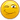Oh no! Where's the JavaScript?

## Lost super admin check box

hi,
we have had a new person join who i would like to become a super admin but i cant find the button anywhere on the administrators screen anymore. I have already changed 3 of us to super admins and require a 4th.(is there a limit to how many are allowed?) i am running v6 with the most current update on it.

<?php
/*---------------------------------------------------+
| PHP-Fusion 6 Content Management System
+----------------------------------------------------+
| http://www.php-fusion.co.uk/
+----------------------------------------------------+
| Released under the terms & conditions of v2 of the
| GNU General Public License. For details refer to
| the included gpl.txt file or visit http://gnu.org
+----------------------------------------------------*/
require_once "../maincore.php";

if (!checkrights("AD"|| !defined("iAUTH"|| \$aid != iAUTH) fallback("../index.php";

\$user_id = isNum(\$_POST['user_id']) ? \$_POST['user_id'] : "0";
\$user_level = isset(\$_POST['make_super']) ? "103" : "102";
if (isset(\$_POST['all_rights']) || isset(\$_POST['make_super'])) {
\$result = dbquery("UPDATE ".\$db_prefix."users SET user_level='\$user_level', user_rights='A.AC.AD.B.C.CP.DB.DC.D.FQ.F.IM.I.IP.M.N.NC.P.PH.PI.PO.S.SL.S1.S2.S3.S4.S5.S6.S7.SU.UG.U.W.WC' WHERE user_id='\$user_id'";
} else {
\$result = dbquery("UPDATE ".\$db_prefix."users SET user_level='\$user_level' WHERE user_id='\$user_id'";
}
}

if (isset(\$remove)) {
if (isNum(\$remove) && \$remove != "1"{
\$result = dbquery("UPDATE ".\$db_prefix."users SET user_level='101', user_rights='' WHERE user_id='\$remove' AND user_level>='102'";
}
}

if (!isNum(\$user_id) || \$user_id == "1"fallback(FUSION_SELF.\$aidlink);
if (isset(\$_POST['rights'])) {
\$user_rights = "";
for (\$i = 0;\$i < count(\$_POST['rights']);\$i++) {
\$user_rights .= stripinput(\$_POST['rights'][\$i]);
if (\$i != (count(\$_POST['rights'])-1)) \$user_rights .= ".";
}
\$result = dbquery("UPDATE ".\$db_prefix."users SET user_rights='\$user_rights' WHERE user_id='\$user_id' AND user_level>='102'";
} else {
\$result = dbquery("UPDATE ".\$db_prefix."users SET user_rights='' WHERE user_id='\$user_id' AND user_level>='102'";
}
}

\$i = 0;
opentable(\$locale['400']);
\$result = dbquery("SELECT * FROM ".\$db_prefix."users WHERE user_level>='102' ORDER BY user_level DESC, user_name";
echo "<table align='center' cellpadding='0' cellspacing='1' width='400' class='tbl-border'>
<tr>
<td class='tbl2'>".\$locale['401']."</td>
<td align='center' width='1%' class='tbl2' style='white-space:nowrap'>".\$locale['402']."</td>
<td align='center' width='1%' class='tbl2' style='white-space:nowrap'>".\$locale['403']."</td>
</tr>\n";
while (\$data = dbarray(\$result)) {
\$row_color = \$i % 2 == 0 ? "tbl1" : "tbl2";
echo "<tr>
<td class='\$row_color'><span title='".(\$data['user_rights'] ? str_replace(".", " ", \$data['user_rights']) : "".\$locale['405'].""."' style='cursor:hand;'>".\$data['user_name']."</span></td>
<td align='center' width='1%' class='\$row_color' style='white-space:nowrap'>".getuserlevel(\$data['user_level'])."</td>
<td align='center' width='1%' class='\$row_color' style='white-space:nowrap'>\n";
if (\$data['user_level'] == "103" && \$userdata['user_id'] == "1"{ \$can_edit = true;
} elseif (\$data['user_level'] != "103"{ \$can_edit = true;
} else { \$can_edit = false; }
if (\$can_edit == true && \$data['user_id'] != "1"{
}
echo "</td>\n</tr>\n";
\$i++;
}
\$result = dbquery("SELECT * FROM ".\$db_prefix."users WHERE user_level<'102' ORDER BY user_name";
if (dbrows(\$result)) {
echo "<tr>
<td align='center' colspan='3' class='tbl1'>
<select name='user_id' class='textbox'>\n";
while (\$data = dbarray(\$result)) {
echo "<option value='".\$data['user_id']."'>".\$data['user_name']."</option>\n";
}
echo "</select>
<input type='checkbox' name='all_rights' value='1'> ".\$locale['411'];
if (\$userdata['user_id'] == "1"echo "<br>\n<input type='checkbox' name='make_super' value='1'> ".\$locale['412'];
echo "\n</form>\n</td>\n</tr>\n";
}
echo "</table>\n";
closetable();
tablebreak();
if (isset(\$edit)) {
if (!isNum(\$edit) || \$edit == "1"fallback(FUSION_SELF.\$aidlink);
\$result = dbquery("SELECT * FROM ".\$db_prefix."users WHERE user_id='\$edit' AND user_level>='102' ORDER BY user_id";
if (dbrows(\$result)) {
\$data = dbarray(\$result);
\$user_rights = explode(".", \$data['user_rights']);
\$result2 = dbquery("SELECT * FROM ".\$db_prefix."admin WHERE admin_page!='4' ORDER BY admin_page ASC,admin_title";
opentable(\$locale['420']);
\$columns = 2; \$counter = 0; \$page = 1;
echo "<table align='center' cellpadding='0' cellspacing='1' width='400' class='tbl-border'>\n";
while (\$data2 = dbarray(\$result2)) {
echo (\$counter % \$columns == 0 ? "</tr>\n" : "<td width='50%' class='tbl1'></td>\n</tr>\n";
\$page++; \$counter = 0;
}
if (\$counter != 0 && (\$counter % \$columns == 0)) echo "</tr>\n<tr>\n";
echo "<td width='50%' class='tbl1'><input type='checkbox' name='rights[]' value='".\$data2['admin_rights']."'".(in_array(\$data2['admin_rights'], \$user_rights) ? " checked" : ""."> ".\$data2['admin_title']."</td>\n";
\$counter++;
}
echo "</tr>
<tr>
<td align='center' colspan='2' class='tbl1'>
<input type='button' class='button' onclick=\"setChecked('rightsform','rights[]',1);\" value='".\$locale['425']."'>
<input type='button' class='button' onclick=\"setChecked('rightsform','rights[]',0);\" value='".\$locale['426']."'>
</td>
</tr>
<tr>
<td align='center' colspan='2' class='tbl1'>
</td>
</tr>
</table>
</form>\n";
closetable();
}
}
echo "<script type='text/javascript'>
function setChecked(frmName,chkName,val){
dml=document.forms[frmName]; len=dml.elements.length;
for(i=0;i<len;i++){if(dml.elements[i].name==chkName){dml.elements[i].checked=val;}}
}
</script>\n";

echo "</td>\n";
require_once BASEDIR."footer.php";
?>

anybody know why? thanks.
Author
Replies
1 post
Views
3,254 times
Last Post
Last updated on 10 years ago